# N-Dimensional Volume Formula

N-dimensional volume generalizes the idea of the space occupied by an object. We can think about N-dimensional volume as being enclosed by N-dimensional vectors.

This post is part of a series.

N-dimensional volume generalizes the idea of the space occupied by an object:

• • 1-dimensional volume refers to the space occupied by a 1-dimensional object, such as the length of a line segment.
• • 2-dimensional volume refers to the space occupied by a 2-dimensional object, such as the area of a square.
• • 3-dimensional volume is what we normally mean by the word "volume" -- the amount of space occupied by a 3-dimensional object, such as the volume of a cube.

Continuing this pattern, we can infer that 4-dimensional volume refers to the space occupied by a 4-dimensional object. It’s harder to come up with an example, though, since it’s difficult to visualize shapes in 4 and higher dimensions.

## Volume Enclosed by N-Dimensional Vectors

However, it becomes easier if we think about N-dimensional volume as being enclosed by N-dimensional vectors.

The length of a unit line segment can be interpreted as the space enclosed by the 1-dimensional unit vector $\left< 1 \right>$.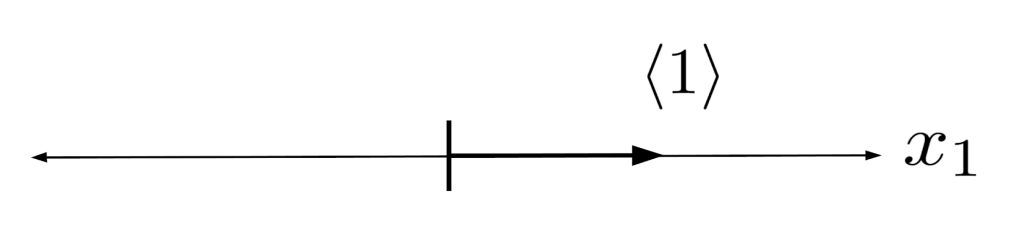The area of a unit square can be interpreted as the space enclosed by the two 2-dimensional vectors $\left< 1,0 \right>$ and $\left< 0,1 \right>$.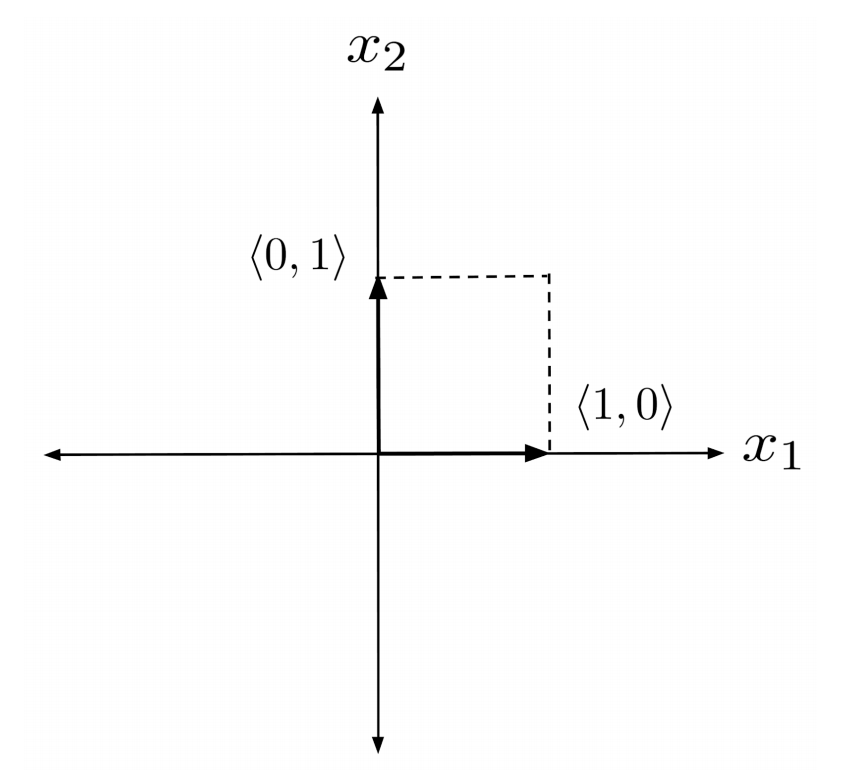The volume of a unit cube can be interpreted as the space enclosed by the three 3-dimensional unit vectors: $\left< 1,0,0 \right>$, $\left< 0,1,0 \right>$, and $\left< 0,0,1 \right>$.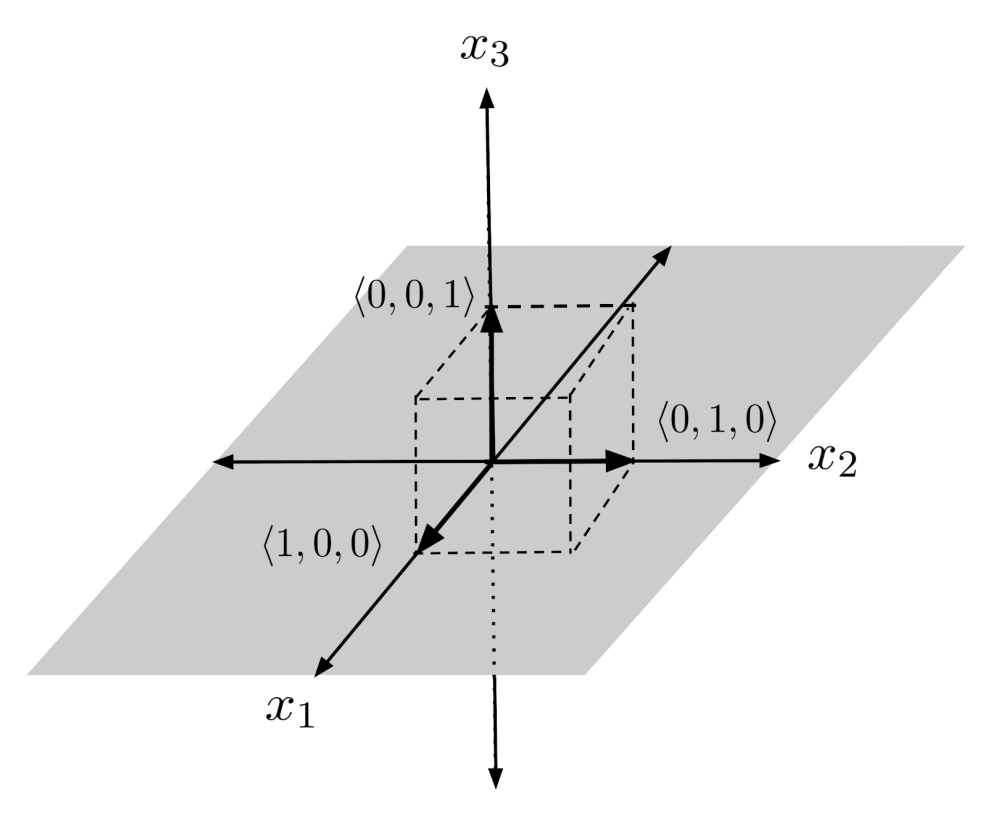Continuing this pattern, the volume of a unit 4-dimensional cube can be interpreted as the space enclosed by the four 4-dimensional unit vectors: $\left< 1,0,0,0 \right>$, $\left< 0,1,0,0 \right>$, $\left< 0,0,1,0 \right>$, and $\left< 0,0,0,1 \right>$.

This is harder to draw, but it gives us a general way to think about volume in N dimensions. The volume of a unit N-dimensional cube can be interpreted as the space enclosed by the N N-dimensional unit vectors:

\begin{align*} \begin{matrix} \left< 1, 0, 0, \cdots, 0,0 \right> \\ \left< 0,1, 0, \cdots, 0,0 \right> \\ \vdots \\ \left<0,0, 0, \cdots, 1, 0 \right> \\ \left<0,0, 0, \cdots, 0,1 \right> \end{matrix} \end{align*}

## Volume of a Parallelogram

Given an object whose sides are perpendicular unit vectors, it’s easy to see that the volume of the object is 1, since the distance of the object in each perpendicular dimension is 1.

But it’s difficult when the vectors are not perpendicular. For example, how would we compute the area of the parallelogram enclosed by the vectors $\left< 4,1 \right>$ and $\left< 2,3 \right>$?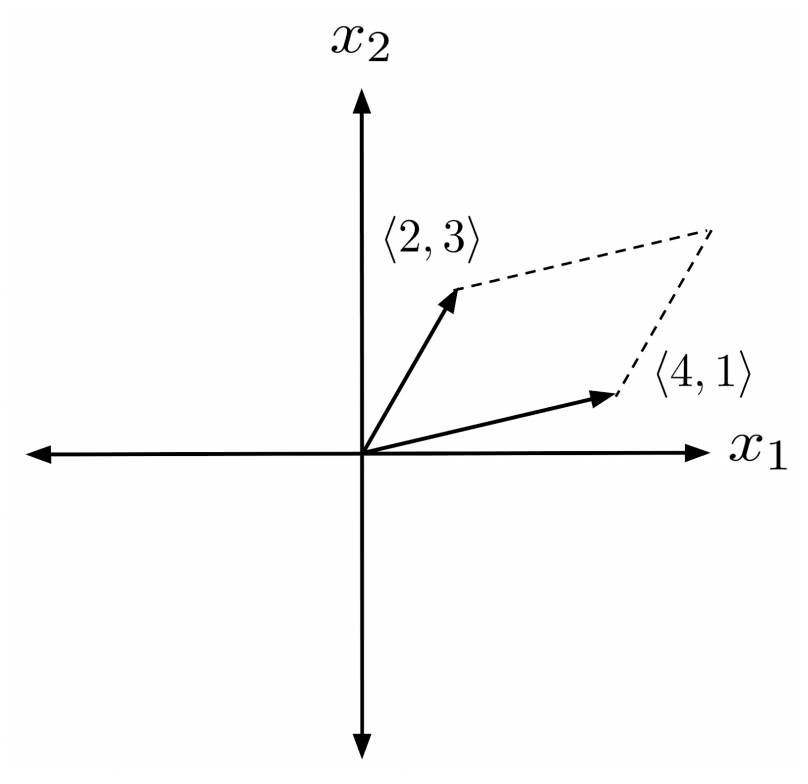Remember, the area of a parallelogram enclosed by two 3-dimensional vectors is just the magnitude of their cross product.

Although the vectors $\left< 4,1 \right>$ and $\left< 2,3 \right>$ are 2-dimensional, we can interpret them as the 3-dimensional vectors $\left< 4,1,0 \right>$ and $\left< 2,3,0 \right>$ in the $x_1 x_2$ plane.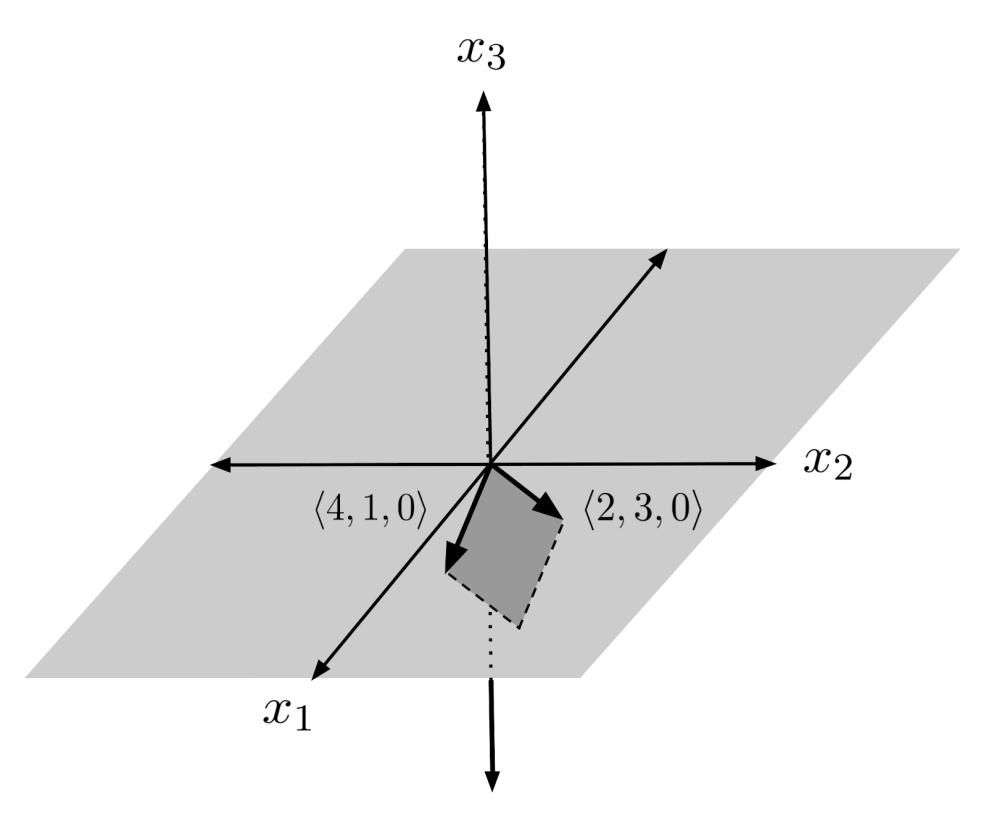Taking the magnitude of the cross product, we find that the area of the parallelogram is $10$.

\begin{align*} \left| \left< 4,1,0 \right> \times \left< 2,3,0 \right> \right| = \left| \left< 0,0,10 \right> \right| = 10 \end{align*}

## Volume of a Parallelepiped

We can also use the cross product as a starting point to find the volume of a parallelepiped enclosed by three 3-dimensional vectors $x$, $y$, and $z$.

The cross product of two of the vectors, say $y \times z$, gives a vector whose magnitude is the area of a face of the parallelepiped, and which points in the direction perpendicular to the face.

The height of the parallelepiped, then, is the length of the remaining vector $x$ in the direction of $y \times z$.

Thus, the volume can be obtained using the dot product:

\begin{align*} x \cdot (y \times z) \end{align*}

This result is known as the triple product.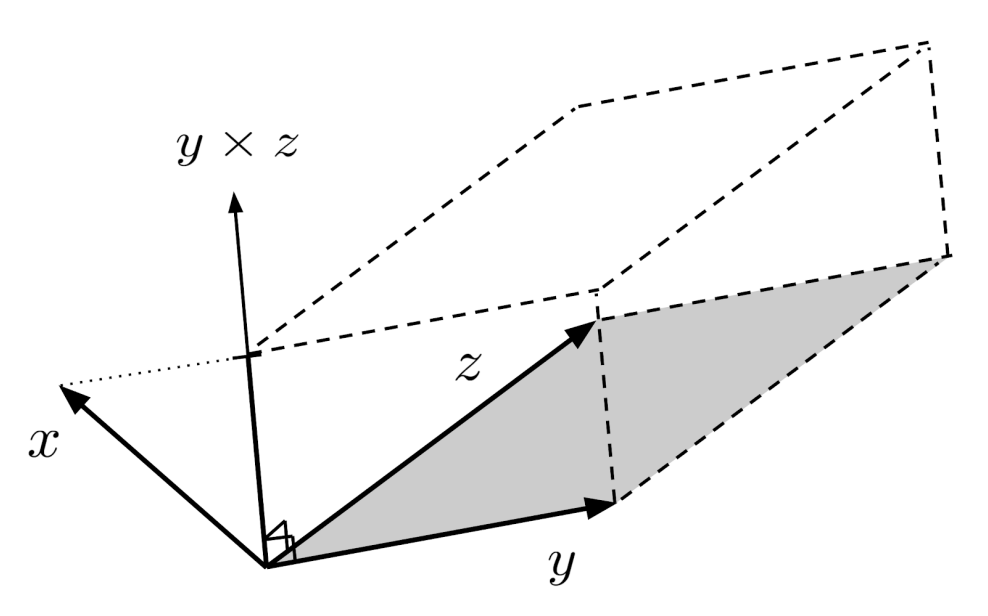That being said, the triple product may come out negative, depending on the order of the vectors in the cross product. So, to compute the volume, we have to take the absolute value of the final result. A simple example for the unit cube is shown below.

\begin{align*} &\left| \left< 1,0,0 \right> \cdot \left( \left< 0,0,1 \right> \times \left< 0,1,0 \right> \right) \right| \\ &= \left| \left< 1,0,0 \right> \cdot \left< -1,0,0 \right> \right| \\ &= \left| -1 \right| \\ &= 1 \end{align*}

Now that we know general methods to compute volume enclosed by 2-dimensional and 3-dimensional vectors, how do we extend this to 4-dimensional vectors?

If we rewrite the 3-dimensional volume formula in terms of the 2-dimensional volume formula, a pattern jumps out at us.

To start, let’s write down the 2-dimensional volume formula for two vectors $x_1 = \left< x_{11}, x_{12} \right>$ and $x_2 = \left< x_{21}, x_{22} \right>$.

\begin{align*} V \begin{pmatrix} \left< x_{11}, x_{12} \right> \\ \left< x_{21}, x_{22} \right> \end{pmatrix} &= \left| \left< x_{11}, x_{12}, 0 \right> \times \left< x_{21}, x_{22}, 0 \right> \right| \\ &= \left| \left< 0,0,x_{11}x_{22} - x_{12}x_{21} \right> \right| \\ &= \left| x_{11}x_{22} - x_{12}x_{21} \right| \end{align*}

However, in order to make the pattern clear, we will leave off the absolute value sign, thereby permitting “signed” volume.

\begin{align*} V \begin{pmatrix} \left< x_{11}, x_{12} \right> \\ \left< x_{21}, x_{22} \right> \end{pmatrix} = x_{11}x_{22} - x_{12}x_{21} \end{align*}

In 2 dimensions, the volume is traced out from the first vector $x_1=\left< x_{11}, x_{12} \right>$ to the second vector $x_2 = \left< x_{21}, x_{22} \right>$, and the sign of the volume just tells us whether the tracing occurs counterclockwise (positive) or clockwise (negative). Intuitively, this convention matches that which is used for tracing out positive or negative angles in the unit circle.

Now, let’s write down the 3-dimensional volume formula for 3 vectors $x_1 = \left< x_{11}, x_{12}, x_{13} \right>$, $x_2 = \left< x_{21}, x_{22}, x_{23} \right>$, and $x_3 = \left< x_{31}, x_{32}, x_{33} \right>$, again leaving off the absolute value sign and thereby permitting “signed” volume. (The meaning of “signed” volume in 3 dimensions will be addressed later.)

\begin{align*} V \begin{pmatrix} \left< x_{11}, x_{12}, x_{13} \right> \\ \left< x_{21}, x_{22}, x_{23} \right> \\ \left< x_{31}, x_{32}, x_{33} \right> \end{pmatrix} &= \left< x_{11}, x_{12}, x_{13} \right> \cdot \left( \left< x_{21}, x_{22}, x_{23} \right> \times \left< x_{31}, x_{32}, x_{33} \right> \right) \\ &= \left< x_{11}, x_{12}, x_{13} \right> \cdot \left( \left< x_{22}x_{33}-x_{23}x_{32}, x_{23}x_{31} -x_{21}x_{33}, x_{21}x_{32} - x_{22}x_{31} \right> \right) \\ &= \left< x_{11}, x_{12}, x_{13} \right> \cdot \left< V \begin{pmatrix} \left< x_{22}, x_{23} \right> \\ \left< x_{32}, x_{33} \right> \end{pmatrix}, V \begin{pmatrix} \left< x_{23}, x_{21} \right> \\ \left< x_{33}, x_{31} \right> \end{pmatrix}, V \begin{pmatrix} \left< x_{21}, x_{22} \right> \\ \left< x_{31}, x_{32} \right> \end{pmatrix} \right> \end{align*}

To ease notation, we define another volume function $V_k$ that also computes volumes of vectors, but first re-indexes the vectors by removing the $\text{k}^\text{th}$ component and moving entries after the $\text{k}^\text{th}$ component to the beginning of the vector. This way, the 3-dimensional volume formula can be simplified to

\begin{align*} V \begin{pmatrix} x_1 \\ x_2 \\ x_3 \end{pmatrix} &= x_1 \cdot \left< V_1 \begin{pmatrix} x_2 \\ x_3 \end{pmatrix}, V_2 \begin{pmatrix} x_2 \\ x_3 \end{pmatrix}, V_3 \begin{pmatrix} x_2 \\ x_3 \end{pmatrix} \right>. \end{align*}

Using this form, we can guess at an N-dimensional volume formula:

\begin{align*} V \begin{pmatrix} x_1 \\ x_2 \\ x_3 \\ \vdots \\ x_N \end{pmatrix} &= x_1 \cdot \left< V_1 \begin{pmatrix} x_2 \\ x_3 \\ \vdots \\ x_N \end{pmatrix}, V_2 \begin{pmatrix} x_2 \\ x_3 \\ \vdots \\ x_N \end{pmatrix}, \ldots, V_N \begin{pmatrix} x_2 \\ x_3 \\ \vdots \\ x_N \end{pmatrix} \right> \end{align*}

## Sanity Checks

Let’s test out this formula on a simple case: the 4-dimensional unit cube, which is enclosed by the vectors $x_1 = \left< 1,0,0,0 \right>$, $x_2 = \left< 0,1,0,0 \right>$, $x_3 = \left< 0,0,1,0 \right>$, and $x_4 = \left< 0,0,0,1 \right>$.

\begin{align*} V \begin{pmatrix} x_1 \\ x_2 \\ x_3 \\ x_4 \end{pmatrix} &= x_1 \cdot \left< V_1 \begin{pmatrix} x_2 \\ x_3 \\ x_4 \end{pmatrix}, V_2 \begin{pmatrix} x_2 \\ x_3 \\ x_4 \end{pmatrix}, V_3 \begin{pmatrix} x_2 \\ x_3 \\ x_4 \end{pmatrix}, V_4 \begin{pmatrix} x_2 \\ x_3 \\ x_4 \end{pmatrix} \right> \\ &= \left< 1,0,0,0 \right> \cdot \left< V_1 \begin{pmatrix} x_2 \\ x_3 \\ x_4 \end{pmatrix}, V_2 \begin{pmatrix} x_2 \\ x_3 \\ x_4 \end{pmatrix}, V_3 \begin{pmatrix} x_2 \\ x_3 \\ x_4 \end{pmatrix}, V_4 \begin{pmatrix} x_2 \\ x_3 \\ x_4 \end{pmatrix} \right> \\ &= V_1 \begin{pmatrix} x_2 \\ x_3 \\ x_4 \end{pmatrix} \\ &= V_1 \begin{pmatrix} \left< 0,1,0,0 \right> \\ \left< 0,0,1,0 \right> \\ \left<0,0,0,1 \right> \end{pmatrix} \\ &= V \begin{pmatrix} \left< 1,0,0 \right> \\ \left< 0,1,0 \right> \\ \left< 0,0,1 \right> \end{pmatrix} \\ &= \left< 1,0,0 \right> \cdot \left( \left< 0,1,0 \right> \times \left< 0,0,1 \right> \right) \\ &= \left< 1,0,0 \right> \cdot \left< 1,0,0 \right> \\ &= 1 \end{align*}

Now we’re reaching the point where it’s hard to actually “see” what’s happening. But the math shows us a pattern, the pattern matches our intuition on a simple case, and given that 3-dimensional volume is the sum of multiples of 2-dimensional volumes, it seems plausible that N-dimensional volume could be the sum of multiples of (N-1)-dimensional volumes.

Moreover, the volume formula matches our intuition when we rescale a vector. Intuitively, rescaling a vector should have the effect of rescaling the volume: for example, if we double the length of one of the sides of a parallelogram, the area should double.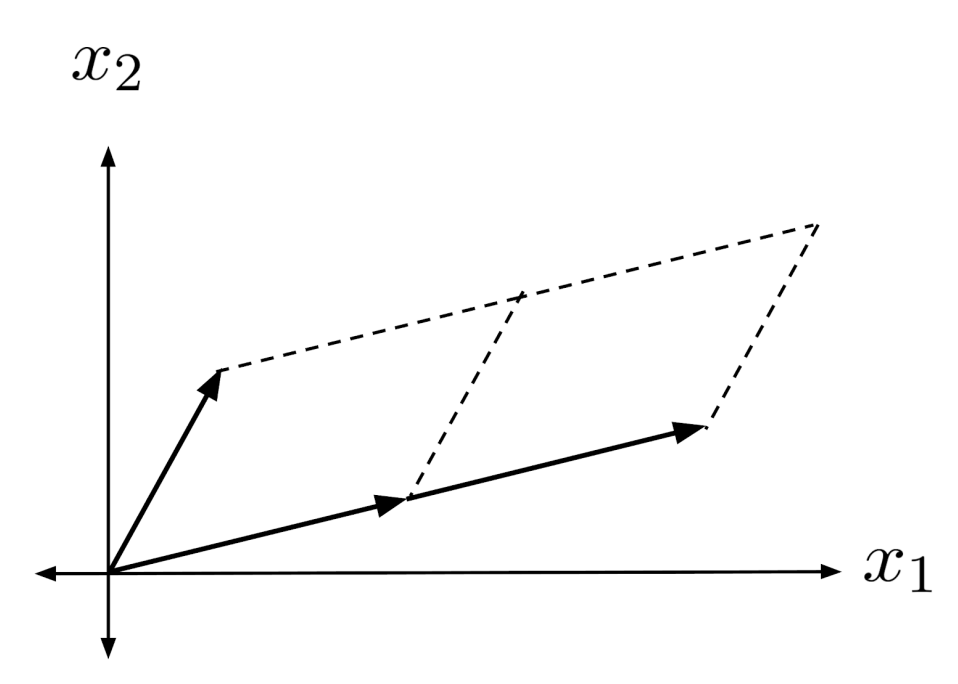Likewise, if we double the length of one of the sides of a parallelepiped, then the volume should double.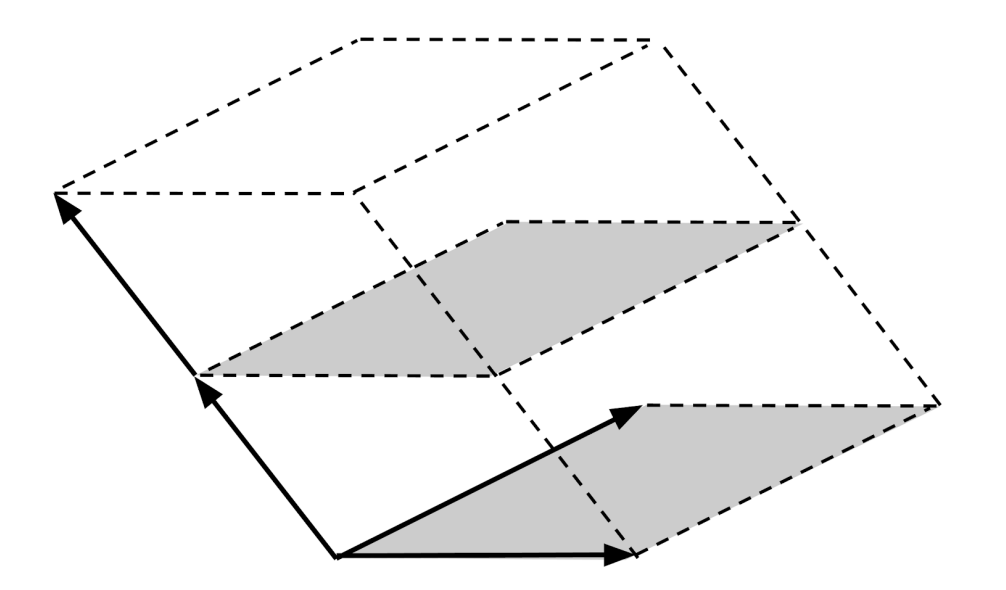More generally, if we rescale a single vector by a factor $r$, then the volume should also be rescaled by a factor $r$. Indeed, this is the case with our formula.

\begin{align*} V \begin{pmatrix} rx_1 \\ x_2 \\ x_3 \\ \vdots \\ x_N \end{pmatrix} &= rx_1 \cdot \left< V_1 \begin{pmatrix} x_2 \\ x_3 \\ \vdots \\ x_N \end{pmatrix}, V_2 \begin{pmatrix} x_2 \\ x_3 \\ \vdots \\ x_N \end{pmatrix}, \ldots, V_N \begin{pmatrix} x_2 \\ x_3 \\ \vdots \\ x_N \end{pmatrix} \right> \\ &= r \left( x_1 \cdot \left< V_1 \begin{pmatrix} x_2 \\ x_3 \\ \vdots \\ x_N \end{pmatrix}, V_2 \begin{pmatrix} x_2 \\ x_3 \\ \vdots \\ x_N \end{pmatrix}, \ldots, V_N \begin{pmatrix} x_2 \\ x_3 \\ \vdots \\ x_N \end{pmatrix} \right> \right) \\ &= r V \begin{pmatrix} x_1 \\ x_2 \\ x_3 \\ \vdots \\ x_N \end{pmatrix} \end{align*}

Of course, we have only shown that rescaling the first vector rescales the volume. However, we can use this fact to show that rescaling the second vector also rescales the volume.

\begin{align*} V \begin{pmatrix} x_1 \\ rx_2 \\ x_3 \\ \vdots \\ x_N \end{pmatrix} &= x_1 \cdot \left< V_1 \begin{pmatrix} rx_2 \\ x_3 \\ \vdots \\ x_N \end{pmatrix}, V_2 \begin{pmatrix} rx_2 \\ x_3 \\ \vdots \\ x_N \end{pmatrix}, \ldots, V_N \begin{pmatrix} rx_2 \\ x_3 \\ \vdots \\ x_N \end{pmatrix} \right> \\ &= x_1 \cdot \left< r V_1 \begin{pmatrix} x_2 \\ x_3 \\ \vdots \\ x_N \end{pmatrix}, r V_2 \begin{pmatrix} x_2 \\ x_3 \\ \vdots \\ x_N \end{pmatrix}, \ldots, r V_N \begin{pmatrix} x_2 \\ x_3 \\ \vdots \\ x_N \end{pmatrix} \right> \\ &= x_1 \cdot r \left< V_1 \begin{pmatrix} x_2 \\ x_3 \\ \vdots \\ x_N \end{pmatrix}, V_2 \begin{pmatrix} x_2 \\ x_3 \\ \vdots \\ x_N \end{pmatrix}, \ldots, V_N \begin{pmatrix} x_2 \\ x_3 \\ \vdots \\ x_N \end{pmatrix} \right> \\ &= r \left( x_1 \cdot \left< V_1 \begin{pmatrix} x_2 \\ x_3 \\ \vdots \\ x_N \end{pmatrix}, V_2 \begin{pmatrix} x_2 \\ x_3 \\ \vdots \\ x_N \end{pmatrix}, \ldots, V_N \begin{pmatrix} x_2 \\ x_3 \\ \vdots \\ x_N \end{pmatrix} \right> \right) \\ &= r V \begin{pmatrix} x_1 \\ x_2 \\ x_3 \\ \vdots \\ x_N \end{pmatrix} \end{align*}

We could keep on going, using similar arguments to show that rescaling the 3rd, 4th, so on, and Nth vector have the same effect of rescaling the volume.

## Final Remarks

Unfortunately, this volume formula is unintuitive and unwieldy for volume computations in high-dimensional space.

Soon, though, we will introduce a more intuitive concept called shearing, which will lead us to an easier, more intuitive process of computing high-dimensional volumes.

The volume formula presented in this chapter is still noteworthy, though, because although shearing will provide us with a process for computing volume, it won’t give us a formula for computing volume.

## Exercises

Compute the N-dimensional unsigned volume $|V(x_1,x_2, \ldots,x_n)|$ enclosed by the vectors $x_1,x_2, \ldots, x_n$. (You can view the solution by clicking on the problem.)

\begin{align*} 1) \hspace{.5cm} x_1 &= \left< 1,0 \right> \\ x_2 &= \left< 0,2 \right> \end{align*}
Solution:
\begin{align*} 2 \end{align*}

\begin{align*} 2) \hspace{.5cm} x_1 &= \left< 1,1 \right> \\ x_2 &= \left< 1,2 \right> \end{align*}
Solution:
\begin{align*} 1 \end{align*}

\begin{align*} 3) \hspace{.5cm} x_1 &= \left< 3,4 \right> \\ x_2 &= \left< -1,2 \right> \end{align*}
Solution:
\begin{align*} 10 \end{align*}

\begin{align*} 4) \hspace{.5cm} x_1 &= \left< 7,-3 \right> \\ x_2 &= \left< 2,-6 \right> \end{align*}
Solution:
\begin{align*} 36 \end{align*}

\begin{align*} 5) \hspace{.5cm} x_1 &= \left< 1,2,3 \right> \\ x_2 &= \left< 3,2,1 \right> \\ x_3 &= \left< 1,3,2 \right> \end{align*}
Solution:
\begin{align*} 12 \end{align*}

\begin{align*} 6) \hspace{.5cm} x_1 &= \left< 2,0,-1 \right> \\ x_2 &= \left< 3,4,2 \right> \\ x_3 &= \left< 0,-1,2 \right> \end{align*}
Solution:
\begin{align*} 23 \end{align*}

\begin{align*} 7) \hspace{.5cm} x_1 &= \left< 1,5,7 \right> \\ x_2 &= \left< 3,2,1 \right> \\ x_3 &= \left< 0,1,2 \right> \end{align*}
Solution:
\begin{align*} 6 \end{align*}

\begin{align*} 8) \hspace{.5cm} x_1 &= \left< 4,0,-5 \right> \\ x_2 &= \left< -9, 1, 7 \right> \\ x_3 &= \left< 2,-5,4 \right> \end{align*}
Solution:
\begin{align*} 59 \end{align*}

\begin{align*} 9) \hspace{.5cm} x_1 &= \left< 1,3,2,0 \right> \\ x_2 &= \left< 4,3,0,1 \right> \\ x_3 &= \left< 0,0,2,1 \right> \\ x_4 &= \left< 3,0,4,2 \right> \end{align*}
Solution:
\begin{align*} 36 \end{align*}

\begin{align*} 10) \hspace{.5cm} x_1 &= \left< 0,-2,3,1 \right> \\ x_2 &= \left< -1,-1,2,2 \right> \\ x_3 &= \left< 1,0,1,0 \right> \\ x_4 &= \left< 0,1,1,1 \right> \end{align*}
Solution:
\begin{align*} 3 \end{align*}

This post is part of a series.

Tags: